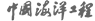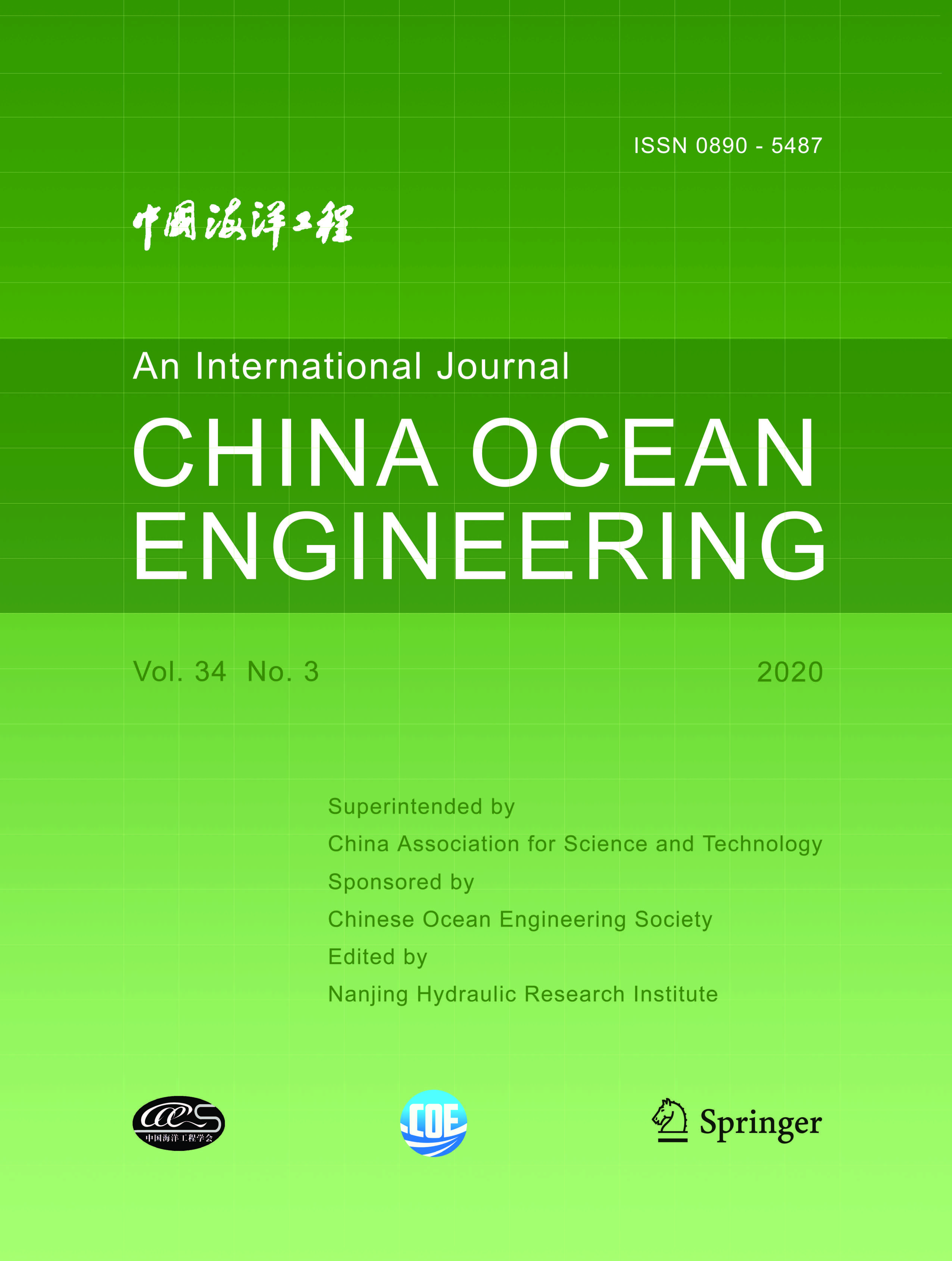ISSN  0890-5487 CN 32-1441/P

## 2004 Vol.(3)

Display Mode：          |

2004, (3): -.
[Abstract](325)
Abstract:
2004, (3): -.
[Abstract](351)
Abstract:
This investigation examines long wave reflection and transmission induced by a sloping step. Bellman and Kalaba's (1959) invariant imbedding is introduced to find wave reflection. An alternative method matching both the surface elevation and its surface slope of each region at the junction is applied to the determination of wave reflection and transmission. The proposed methods are compared with the accurate numerical results of Porter and Porter (2000) and those of Mei (1983) for a vertical step. The wave reflection obtained for a mildly sloping step differs significantly from the result of Mei. The wave reflection is found to fluctuate owing to wave trapping for the mild sloping step. The height and the face slope of the step are important for determining wave reflection and transmission coefficients.
2004, (3): -.
[Abstract](502)
Abstract:
2004, (3): -.
[Abstract](498)
Abstract:
The model tests are performed with regular waves, and the effect of wave height, wave period, water depth, sediment size and pile diameter is evaluated. The shape and size of local scour around piles are studied. There are three typical scour patterns due to wave action. It is found that a relationship exists between the erosion depth and the wave number. An empirical formula of the maximum local scour is thus derived.
2004, (3): -.
[Abstract](491)
Abstract:
2004, (3): -.
[Abstract](536)
Abstract:
Considering the effect of the internal flowing fluid and the external marine environmental condition, the differential equation for the vortex induced vibration (VIV) of the free spanning pipeline is derived and is discretized by the Hermit interpolation function. The free vibration equation with the damping term is solved by the complex damping method for the natural frequency, and then the effect of fluid damping on the natural frequency of the free spanning pipeline is analyzed. The results show that fluid damping has a significant influence on the damped natural frequency of the free spanning pipeline in the lock in state, while it has little influence when the pipeline is out of the lock in state. In the meantime, the change of the free span length has the same effect on the damped natural frequency and the undamped natural frequency.
2004, (3): -.
[Abstract](325)
Abstract:
This paper presents a numerical model study of the propagation of water waves using the parabolic approximation of the mild slope equation in the orthogonal coordinate system. Two types of coordinate systems are studied: (a) a general form of orthogonal coordinate system and (b) the conformal system, a special form of orthogonal coordinate system. Two typical examples, namely, expanded breakwaters and a circular channel, are studied to validate the model. First, the examples are studied by use of the general orthogonal coordinates. Then the same examples are computed by use of the conformal system. The computational results show that the conformal coordinate system generally gives better predictions than the general orthogonal system. A numerical technique for generating the conformal grid is combined with the numerical model to improve the practicability of the model. The comparison between the result from the numerical grid system and that from the analytical grid system shows that reliable computational results can be obtained by use of the numerical conformal grid system.
2004, (3): -.
[Abstract](331)
Abstract:
Large groundwater table fluctuations were observed in a coastal aquifer during an offshore storm. The storm induced significant changes of the mean shoreline elevation, characterized by a pulse like oscillation. This pulse propagated in the aquifer, resulting in the water table fluctuations. A general analytical solution is derived to quantify this new mechanism of water table fluctuation. The solution is applied to field observations and is found to be able to predict reasonably well the observed storm induced water table fluctuations. Based on the analytical solution, the damping characteristics and phase shift of the oscillation as it propagates inland are examined.
2004, (3): -.
[Abstract](325)
Abstract:
In this paper, a numerical model is established for estimating the wave forces on a submerged horizontal circular cylinder. For predicting the wave motion, a set of two dimensional Navier Stokes equations is solved numerically with a finite element method. In order to track the moving non linear wave surface boundary, the Navier Stokes equations are discretized in a moving mesh system. After each computational time step, the mesh is modified according to the changed wave surface boundary. In order to stabilize the numerical procedure, a three step finite element method is applied in the time integration. The water sloshing in a tank and wave propagation over a submerged bar are simulated for the first time to validate the present model. The computational results agree well with the analytical solution and the experimental data. Finally, the model is applied to the simulation of interaction between waves and a submerged horizontal circular cylinder. The effects of the KC number and the cylinder depth on the wave forces are studied.
2004, (3): -.
[Abstract](316)
Abstract:
2004, (3): -.
[Abstract](320)
Abstract:
2004, (3): -.
[Abstract](326)
Abstract:
2004, (3): -.
[Abstract](333)
Abstract:
In the paper, a comprehensive numerical study on the moored system is performed in time domain. The moored system, which is composed of the floating body sub system and the mooring line sub system, is calculated as a whole system by coupling. A time domain method is applied to the analysis of the mooring line sub system, and at the same time, an indirect time domain method translated from frequency domain to time domain is developed to calculate the floating body sub system. In the end, an FPSO vessel is calculated as a numerical example by the present method. A comparison of the result of the model test and that of the numerical method indicates that the present method is exact and effective.
2004, (3): -.
[Abstract](328)
Abstract:
Investigated in this study is the flow induced vibration of a nonlinearly restrained curved pipe conveying fluid. The nonlinear equation of motion is derived by equilibrium of forces on microelement of the system under consideration. The spatial coordinate of the system is discretized by DQM (differential quadrature method). On the basis of the boundary conditions, the dynamic equation is solved by the Newton Raphson iteration method. The numerical solutions reveal several complex dynamic motions for the variation of the fluid velocity parameter, such as limit cycle motion, buckling and so on. The result obtained also shows that the sub parameter regions corresponding to the several motions may change with the variation of some parameters of the curved pipe. The present study supplies a new reference for investigating the nonlinear dynamic response of some other structures.
2004, (3): -.
[Abstract](343)
Abstract:
The paper contains the results of an experimental study on a planing catamaran. The aim of this study is resistance reduction with application of foils. Experiments are performed in different conditions and the results are compared with each other. The foils are used in different configurations and it is concluded that unsuitable design may result in larger resistance. But, it is also shown that, for a good design, the resistance may be reduced considerably.
2004, (3): -.
[Abstract](329)
Abstract:
More and more researches show that neither the critical downward acceleration nor the critical slope of water waves is a universal constant. On the contrary, they vary with particular wave conditions. This fact renders the models either for the probability of wave breaking B or for the whitecap coverage W based on these criteria difficult to apply. In this paper and the one which follows we seek to develop models for the prediction of both B and W based on the kinematical criterion. First, several joint probabilistic distribution functions (PDFs) of wave characteristics are derived, based on which the breaking properties B and W are estimated. The estimation is made on the assumption that a wave breaks if the horizontal velocity of water particles at its crest exceeds the local wave celerity, and whitecapping occurs in regions of fluid where water particles travel faster than the waves. The consequent B and W depend on wave spectral moments of orders 0 to 4. Then the JONSWAP spectrum is used to represent the fetch limited sea waves in deep water, so as to relate the probability of wave breaking and the whitecap coverage with wind parameters. To this end, the time averaging technique proposed by Glazman (1986) is applied to the estimation of the spectral moments involved, and furthermore, the theoretical models are compared with available observations collected from published literature. From the comparison, the averaging time scale is determined. The final models show that the probability of wave breaking as well as the whitecap coverage depends on the dimensionless fetch. The agreement between these models and the database is reasonable.
2004, (3): -.
[Abstract](323)
Abstract:
Experiments were made on plain concrete subjected to triaxial static loading and constant amplitude compressive fatigue loading with a constant lateral pressure in two directions. The initial confining pressure was 0, 0.1 f c , 0.25 f c and 0.4 f c , respectively, for the static test, and 0.1 f c and 0.25 f c for the fatigue test. Based on the triaxial compressive constitutive behavior of concrete, the inflexion of confining pressure evolution was chosen to be the fatigue damage criterion during the test. The rule of evolution of longitudinal maximum and minimum strains, longitudinal cyclic modulus and damage were recorded and analyzed. According to the Fardis Chen criterion model and the concept of equivalent fatigue life and equivalent stress level, a unified S N curve for multi axial compressive fatigue loading was proposed. Thus, the fatigue strength factors for different fatigue loading cases can be obtained. The present investigation provides information for the fatigue design of concrete structures.
2004, (3): -.
[Abstract](352)
Abstract:
Based on the second order random wave solutions of water wave equations in finite water depth, statistical distributions of the depth integrated local horizontal momentum components are derived by use of the characteristic function expansion method. The parameters involved in the distributions can be all determined by the water depth and the wave number spectrum of ocean waves. As an illustrative example, a fully developed wind generated sea is considered and the parameters are calculated for typical wind speeds and water depths by means of the Donelan and Pierson spectrum. The effects of nonlinearity and water depth on the distributions are also investigated.

### Current Issue1. Editor-in-Chief:

2. Superintended by:

CHINA ASSOCIATION FOR SCIENCE AND TECHNOLOGY

Chinese Ocean Engineering Society （COES）

4. Edited by:

Nanjing Hydraulic Research InstituteAddress: 34 Hujuguan, Nanjing 210024, China Pos: 210024 Tel: 025-85829388 E-mail: coe@nhri.cn

Support by Beijing Renhe Information Technology Co. Ltd E-mail: info@rhhz.net